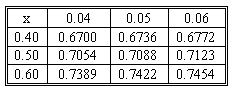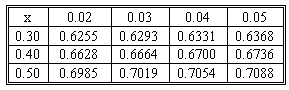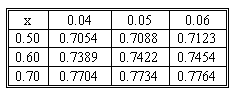### Why should I choose AnalystNotes?

Simply put: AnalystNotes offers the best value and the best product available to help you pass your exams.

### Subject 6. The Black-Scholes-Merton model

Assumptions of the Black-Scholes-Merton Model:

• The underlying price follows a lognormal probability distribution as it evolves through time.

A lognormal probability distribution is one in which the log return is normally distributed. For example, if a stock moves from 100 to 110, the return is 10% but the log return is ln(1.10) = 0.0953 or 9.53%. This assumption is reasonable for most assets that offer options. Additionally, the variance of the return is assumed to be constant for the life of the option.

• Interest rates remain constant and known.

The model uses the risk-free rate to represent this constant and known rate. During periods of rapidly changing interest rates, these 30 day rates are often subject to change, thereby violating one of the assumptions of the model. The assumption also becomes a problem for pricing options on bonds and interest rates.

• The volatility of the underlying asset is known and constant.

The volatility of the underlying is specified in the form of the standard deviation of the log return. This is the most critical assumption.

• No transaction costs or taxes.

• No cash flows on the underlying.

• European exercise terms are used.

This limitation is not a major concern because very few calls are ever exercised before the last few days of their life. This is true because when you exercise a call early, you forfeit the remaining time value on the call and collect the intrinsic value. Towards the end of the life of a call, the remaining time value is very small, but the intrinsic value is the same.

The Black-Scholes-Merton formulas for the prices of call and put options are:

c = S0 N(d1) - X e -rcT N(d2)
p = X e -rc T [1 - N(d2)] - S0[1 - N(d1)]

where:
d1 = {ln(S0/X) + [rc + (σ2/2)] T} / [σ (T1/2)].
d2 = d1 - σ (T1/2).
σ = the annualized standard deviation of the continuously compounded return on the stock.
rc = the continuously compounded risk-free rate of return.
N(.) = cumulative normal distribution function.

Assume that a stock trades at \$100 and the continuously compounding risk-free interest rate is 6%. A call option on the stock has an exercise price of \$100 and expires in one year. The standard deviation of the stock's returns is 0.1 per year. We compute the values of d1 and d2 as follows:
d1 = [ln(100/100) + (0.06 + (0.12/2) 1) / (0.1 11/2) = 0.65.
d2 = 0.65 - 0.1 x 11/2 = 0.55.

Next, we find the cumulative normal values associated with d1 and d2. These values are the probability that a normally distributed variable with a zero mean and a standard deviation of 1.0 will have a value equal to or less than the d1 or d2 term we are considering. Below is the relevant part of the cumulative probabilities table for a standard normal distribution.For a value of 0.65 drawn from the border of the table, we find our probability in the interior: N(d1) = N(0.65) = 0.7422. Similarly, N(d2) = N(0.55) = 0.7088. We now have:
c = \$100 x 0.7422 - \$100 x e -0.06 x 1 x 0.7088 = \$7.46.
p = \$100 x e -0.06 x 1 x (1 - 0.7088) - \$100 x (1 - 0.7422) = \$1.64.

Inputs to the Black-Scholes-Merton model

• The underlying price:

See subject g for details.

• The exercise price:

This input does not change for an option. However, we may want to see how it affects the option price if we choose an option with a different exercise price. As we have previously seen, the call option price will be lower the higher the exercise price, and the put option price will be higher.

• The risk-free rate:

The sensitivity of the option price to the risk-free rate is called the rho. Although the model assumes a constant risk-free rate, we can still explore how the option price would differ if the current rate were different.

• Large changes in the interest rate have relatively little impact on the option prices. That is, the price of a European option on an asset is not very sensitive to the risk-free rate.

• For a call option, rho (RHOc) is always positive. For a put option, rho (RHOp ) is always negative.

• RHOc and RHOp change as time passes, with both tending toward zero as expiration approaches. The interest rate affects the price of an option in conjunction with the time remaining until expiration mainly through the time value of money. If little time remains until expiration, the interest rate is relatively unimportant, and the price of an option becomes less sensitive to the interest rate.

• Time to expiration:

If the stock price and all other parameters of the option pricing model remain constant, the price of options will still change with the passage of time. Theta is the negative of the first derivative of the option price with respect to the time remaining until expiration. THETAc and THETAp are generally less than 0.

The tendency for option prices to change due merely to the passage of time is known as time decay. If all parameters remain constant, except the expiration date draws nearer, a call or a put option will have to fall in value, and the theta will be negative. It will be worthless at expiration as S = X. Therefore, a call or a put option will lose its entire value through time decay.

• Volatility:

Volatility is the standard deviation of the continuously compounded return on the stock. It is the only variable that cannot be obtained easily and directly from another source.

Option prices are extremely sensitive to the volatility. Vega is the first derivative of an option's price with respect to the volatility of the underlying stock.

• VEGAc and VEGAp are identical and always positive. This means that if the volatility increases, both call and put prices increase.

• Vega tends to be greatest for an option near-the-money. When an option is deep-in-the-money or deep-out-of-the-money, the vega is low and can approach zero.

#### Practice Question 1

Consider a stock that trades for \$75. A call on this stock has an exercise price of \$70 and it expires in 150 days. If the continuously compounding interest rate is 7% and the standard deviation for the stock's return is 0.35, compute the price of the call option according to Black-Scholes-Merton model.

Below is the relevant part of the cumulative probabilities table for a standard normal distribution.d1 = {ln(75/70) + [0.07 + (0.352/2)] x (150/365)} / (0.35 (150/365)1/2) = 0.5479.
d2 = 0.5479 - 0.35 x (150/365)1/2 = 0.3235.
N(d1) = N(0.55) = 0.7088.
N(d2) = N(0.32) = 0.6255.
c = \$75 x 0.7088 - \$70 x e -0.07 x (150/365) x 0.6255 = \$10.62.

#### Practice Question 2

Consider a stock that trades for \$75. A put on this stock has an exercise price of \$70 and it expires in 150 days. If the continuously compounding interest rate is 7% and the standard deviation for the stocks return is 0.35, compute the price of the put option according to Black-Scholes-Merton model.

Below is the relevant part of the cumulative probabilities table for a standard normal distribution.d1 = {ln(75/70) + [0.07 + (0.352/2)] x (150/365)} / (0.35 (150/365)1/2) = 0.5479.
d2 = 0.5479 - 0.35 x (150/365)1/2 = 0.3235.
N(d1) = N(0.55) = 0.7088.
N(d2) = N(0.32) = 0.6255.
p = \$70 x e -0.07 x (150/365) x (1 - 0.6255) - \$75 x (1 - 0.7088) = \$3.63.

#### Practice Question 3

When an option is near-the-money:

I. VEGA is larger than the VEGA when the option is deep-in-the-money.
II. VEGA is larger than the VEGA when the option is deep-out-of-the-money.

#### Practice Question 4

For a call option:

I. RHO is always negative.
II. THETA is generally negative.
III. VEGA is always negative.

For a call option, rho (RHOc) is always positive. For a put option, rho (RHOp ) is always negative.
VEGAc and VEGAp are identical and always positive.

#### Practice Question 5

As expiration approaches,

I. RHOc tends to be 0.
II. RHOp tends to be 0.
III. THETAc will be positive.
IV. THETAp will be negative.
V. VEGAc will be positive.
VI. VEGAp will be positive.

Correct Answer: I, II, IV, V, VI

RHOc and RHOp change as time passes, with both tending toward zero as expiration approaches. Therefore I and II are correct.
The theta will be negative as the expiration date draws nearer. Therefore IV is correct.
VEGA is always positive for both calls and puts, so V and VI are correct.

#### Practice Question 6

A stock is selling for \$69.88. The risk-free rate is 5%.

Consider the following options on this stock:
Put option A: Exercise price X = \$70, Days to option expiration = 15.
Put option B: Exercise price X = \$70, Days to option expiration = 120.
Call option C: Exercise price X = \$70, Days to option expiration = 15.
Call option D: Exercise price X = \$70, Days to option expiration = 120.

Which statement(s) is (are) true?

I. RHOA > RHOB.
II. RHOA < RHOB.
III. RHOC > RHOD.
IV. RHOC < RHOD.

For a call option, rho (RHOc) is always positive. For a put option, rho (RHOp ) is always negative. RHOc and RHOp change as time passes, with both tending toward zero as expiration approaches.

#### Practice Question 7

A stock sells for \$110. A call option on the stock has an exercise price of \$105 and expires in 43 days. If the continuously compounding interest rate is 0.11 and the standard deviation of the stock's returns is 0.25, what is the price of the call option according to Black-Scholes-Merton model?

Below is the relevant part of the cumulative probabilities table for a standard normal distribution.A. 6.95.
B. 7.82.
C. 8.08.

d1 = {ln(110/105) + [0.11 + (0.252/2)] x (43/365)} / (0.25 (43/365)1/2) = 0.7361.
d2 = 0.7361 - 0.25 x (43/365)1/2 = 0.6503.
N(d1) = N(0.74) = 0.7704.
N(d2) = N(0.65) = 0.7422.
c = \$110 x 0.7704 - \$105 x e -0.11 x (43/365) x 0.7422 = \$7.82.

#### Practice Question 8

A stock sells for \$110. A put option on the stock has an exercise price of \$105 and expires in 43 days. If the continuously compounding interest rate is 0.11 and the standard deviation of the stock's returns is 0.25, what is the price of the put option according to Black-Scholes-Merton model?

Below is the relevant part of the cumulative probabilities table for a standard normal distribution.A. 1.46.
B. 2.32.
C. 2.78.

d1 = {ln(110/105) + [0.11 + (0.252/2)] x (43/365)} / (0.25 (43/365)1/2) = 0.7361.
d2 = 0.7361 - 0.25 x (43/365)1/2 = 0.6503.
N(d1) = N(0.74) = 0.7704.
N(d2) = N(0.65) = 0.7422.
p = \$105 x e -0.11 x (43/365) x (1 - 0.7422) - \$110 x (1 - 0.7704) = \$1.46.

#### Practice Question 9

A stock is selling for \$69.88. The risk-free rate is 5%.

Consider the following call options on this stock:
Call option A: Exercise price X = \$70, Days to option expiration = 120.
Call option B: Exercise price X = \$75, Days to option expiration = 120.

Which statement(s) is (are) true?

I. VEGAA > VEGAB.
II. VEGAA < VEGAB.
III. GAMMAA > GAMMAB.
IV. GAMMAA < GAMMAB.

A. I and III
B. II and III
C. I and IV

Note that option A is near-the-money. Vega tends to be greatest for an option near-the-money so I is correct.

Gamma is large when the option is near-the-money so III is correct.

#### Practice Question 10

______ measures the change of option price with respect to the time remaining until expiration.

A. THETA.
B. RHO.
C. GAMMA.

Theta is the negative of the first derivative of the option price with respect to the time remaining until expiration.

#### Practice Question 11

According to the Black-Scholes option valuation formula, which of the following outcomes is likely as a result of an increase in the price volatility of an underlying asset?

A. The premium of both a call option and a put option will increase.
B. Only the call premium will increase while the put premium will decrease.
C. Only the out premium will increase while the call premium will decrease.

The greater the volatility of the underlying asset, the more valuable will be option, whether it be a call or a put. Options are rights, meaning that the holder will only exercise if the market moves in her favor. Therefore, the greater the volatility, the more the markets could move in the favor of the option holder. Should the market move against the option holder's favor, the most that could be lost is the premium paid for the option.

#### Practice Question 12

Which of the following statements is (are) true with respect to valuing contracts?

I. The time value of both a call and a put will decrease as the contracts near expiration.
II. For a call to be at the money, its intrinsic value must be greater than zero.
III. Due to the efficiency of the option's markets, the actual price of the option is usually equal to its intrinsic value.
IV. For a put contract to be out of the money, the actual price of the underlying asset must be greater than the strike price of the option.

A. I, II, and III
B. I and IV
C. II, III, and IV

II is incorrect because for a call to be at the money, its intrinsic value must also be equal to zero.

III is incorrect because even though the option markets are extremely efficient, the actual price of the option contracts is equal to the sum of the option's intrinsic value and its time value. Only on the expiration date will the actual price of the option be equal to its intrinsic value.

#### Practice Question 13

Which of the following is(are) true with respect to option Vegas?

I. As the volatility of the underlying asset increases, a call option will increase in value while a put option will decrease in value.
II. Vega for a call option is at its highest when the call is at-the-money.
III. For call options and put options that share the same parameters, their Vegas will be the same.

IV. Vega for a put option increases as the option move deeper out-of-the-money.

A. II and IV
B. II and III
C. I, II and IV